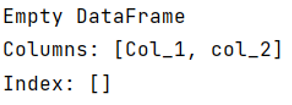# How to create an empty DataFrame with only column names?

Here, we have to create an empty DataFrame with only column names.
Submitted by Pranit Sharma, on May 19, 2022

DataFrames are 2-dimensional data structures in pandas. DataFrames consist of rows, columns, and the data. DataFrame can be created with the help of python dictionaries, here we are creating an empty DataFrame with only column names.

On the other hand, Columns are the different fields that contains their particular values when we create a DataFrame. We can perform certain operations on both rows & column values.

To work with pandas, we need to import pandas package first, below is the syntax:

```import pandas as pd
```

Let us understand with the help of an example.

```# Importing Pandas package
import pandas as pd

# Create a DataFrame
df = pd.DataFrame({'Col_1':[], 'col_2':[]})

# Display DataFrame
print(df)
```

Output:Preparation

What's New

Top Interview Coding Problems/Challenges!